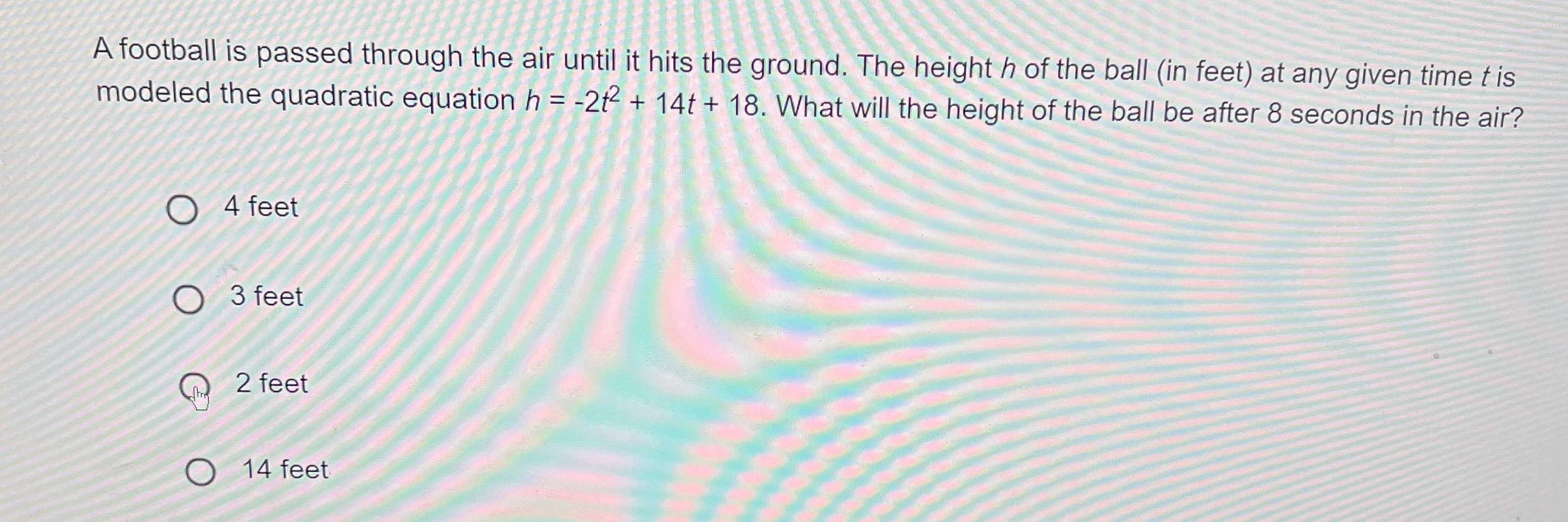### ¿Todavía tienes preguntas de matemáticas?

Pregunte a nuestros tutores expertos
Algebra
PreguntaA football is passed through the air until it hits the ground. The height $$h$$ of the ball (in feet) at any given time $$t$$ is modeled the quadratic equation $$h = - 2 t ^ { 2 } + 14 t + 18$$ . What will the height of the ball be after $$8$$ seconds in the air?

$$4$$ feet

$$3$$ feet

$$2$$ feet

$$14$$ feet

$$h(8) = 2 \space feet$$